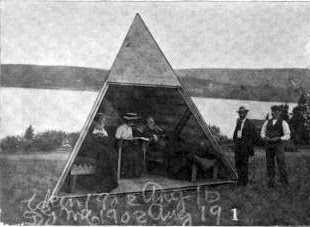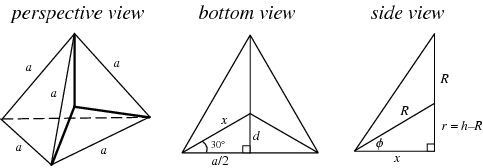### The Graham Bell's tetrahedronal shed

Tony Smith realized an interesting shed that it seems inspired by the tetrahedron, a particular polyhedron, but following Tropolism, this idea was just used by Alexander Graham Bell:The tetrahedron is in general a polyhedron constituted by four triangular sides. Now, if we describe every vertices of every sides with the vector $(x_i, y_i, z_i)$, where $i = 1, \cdots, 4$, then the volume of the tetrahedron is given by: $V = \frac{1}{3!} \begin{vmatrix} x_1 & y_1 & z_1 & 1\\ x_2 & y_2 & z_2 & 1\\ x_3 & y_3 & z_3 & 1\\ x_4 & y_4 & z_4 & 1 \end{vmatrix}$ And if the tetrahedron is regular, we can relate in one beutiful formula, the volume $V$, the area $\Delta$ of the triangles and the radius $R$ of the sphere outside the tetrahedron (or the circumsphere)(1, *) $6RV = \Delta^2$ The regulartetrahedron is also the platonic solid $P_5$
(...) with four polyhedron vertices, six polyhedron edges, and four equivalent equilateral triangular faces.
His symmetries are a bit complex, with 12 rotational symmetries, and the tetrahedral group is isomorphic with the symmetric group $S_4$, i.e. the group of all permutations of 4 elements.The tetrahedron is also the basic idea to the Four corner project, developed by the artist David Barr in 1976, with the idea to realize a Erth-size tetrahedron in order to span our planet.
Finally we can find tetrahedron also in chemistry: methane, xenon tetroxide, perchlorate ion, sulfate ion, phosphate ion and others.
It's also interesting observe that also water presents a structure like a tetrahedron, but in this case isn't a regular polyhedron. In particular:
The most common arrangement of liquid water molecules is tetrahedral with two hydrogen atoms covalently attached to oxygen and two attached by hydrogen bonds. Since the hydrogen bonds vary in length many of these water molecules are not symmetrical and form transient irregular tetrahedra between their four associated hydrogen atoms.(2, 3)

(1) MathWorld: Weisstein, Eric W. Tetrahedron; Jackson, Frank and Weisstein, Eric W. Regular Tetrahedron
(2) Wikipedia: Tetrahedral molecular geometry
(3) P. E. Mason and J. W. Brady (2007). "Tetrahedrality" and the Relationship between Collective Structure and Radial Distribution Functions in Liquid Water. J. Phys. Chem. B 111 (20): 5669–5679
(*) Following wereatheist (read comments) I try to verify the following formula (that I print in the first version of this post): $6RV = \Delta$ I don't find nothing of useful in the following paper:
Staudt , K.G.C. von (1860). Ueber einige geometrische Sätze. Journal für die reine und angewandte Mathematik, 57, 88-89 DOI: 10.1515/crll.1860.57.88 (free version on Gottinger Digitalisierungszentrum)
So I decided to trust to the dimensional control and I changed the formula!

1.Without looking it up, but shouldn´t the formula
1.